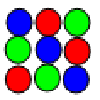Search by Topic

Resources tagged with Modular arithmetic similar to Remainders:

Filter by: Content type:
Age range:
Challenge level:

There are 13 results

Broad Topics > Numbers and the Number System > Modular arithmeticTwo Much

Age 11 to 14 Challenge Level:

Explain why the arithmetic sequence 1, 14, 27, 40, ... contains many terms of the form 222...2 where only the digit 2 appears.What Numbers Can We Make Now?

Age 11 to 14 Challenge Level:

Imagine we have four bags containing numbers from a sequence. What numbers can we make now?A One in Seven Chance

Age 11 to 14 Challenge Level:

What is the remainder when 2^{164}is divided by 7?Differences

Age 11 to 14 Challenge Level:

Can you guarantee that, for any three numbers you choose, the product of their differences will always be an even number?Elevenses

Age 11 to 14 Challenge Level:

How many pairs of numbers can you find that add up to a multiple of 11? Do you notice anything interesting about your results?Going Round in Circles

Age 11 to 14 Challenge Level:

Mathematicians are always looking for efficient methods for solving problems. How efficient can you be?What Numbers Can We Make?

Age 11 to 14 Challenge Level:

Imagine we have four bags containing a large number of 1s, 4s, 7s and 10s. What numbers can we make?How Much Can We Spend?

Age 11 to 14 Challenge Level:

A country has decided to have just two different coins, 3z and 5z coins. Which totals can be made? Is there a largest total that cannot be made? How do you know?Days and Dates

Age 11 to 14 Challenge Level:

Investigate how you can work out what day of the week your birthday will be on next year, and the year after...Where Can We Visit?

Age 11 to 14 Challenge Level:

Charlie and Abi put a counter on 42. They wondered if they could visit all the other numbers on their 1-100 board, moving the counter using just these two operations: x2 and -5. What do you think?Latin Squares

Age 11 to 18

A Latin square of order n is an array of n symbols in which each symbol occurs exactly once in each row and exactly once in each column.The Best Card Trick?

Age 11 to 16 Challenge Level:

Time for a little mathemagic! Choose any five cards from a pack and show four of them to your partner. How can they work out the fifth?It Must Be 2000

Age 7 to 11 Challenge Level:

Here are many ideas for you to investigate - all linked with the number 2000.